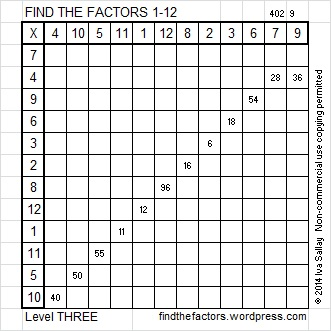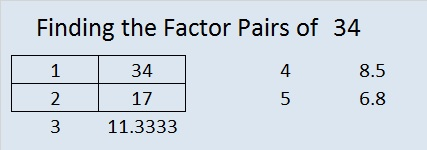# 34 and Step by Step Level 3

Level 3 puzzles are designed to be solved starting from a row or column at the top of the puzzle with 2 clues. First, find the common factor of those two clues that will allow you to write only numbers from 1 to 10 in the first column of the puzzle. Then factor row by row to the bottom of the puzzle making sure each number from 1 to 10 is written only once in both the factor row and the factor column. You will notice a rhythm for the answers as you work.This week’s puzzles are also available in an excel file here. If you have a spreadsheet program on your computer, you can access it. If you enable editing in excel, you can type your answers directly onto the puzzle, and you can also easily print the puzzles.

Here is the solution to last week’s level 3 puzzle:Since level 3 puzzles are designed to be solved from the top of the puzzle to the bottom, they are fairly easy to solve. I will not usually write step by step instructions for them, but I will today.

Last week’s puzzle was solved by beginning with the clues 28 and 36 because they are both in the same row. Their common factors are 1, 2, and 4. Choose 4 because it is the only common factor that will allow us to write only numbers 10 or less in the factor row. Below is a chart showing the steps IN ORDER that were used to solve this puzzle using logic. The chart states what to write in the factor column and the factor row for each clue.Just as a Find the Factor puzzle can be solved step by step, so can a 4 x 4 Magic Square:

34 is the magic sum of a 4 x 4 magic square. There are 880 ways times 8 different orientations to make a 4 x 4 square. I made a gif that shows one way. Look and you will see that half the numbers are in the correct positions right from the start:

To find other 4 x 4 magic squares, try this method.

34 is a composite number. 34 = 1 x 34 or 2 x 17. Factors of 34: 1, 2, 17, 34. Prime factorization: 34 = 2 x 17.34 is never a clue in the FIND THE FACTORS puzzles.

## One thought on “34 and Step by Step Level 3”

1. Pingback: nebusresearch | Factor Finding

This site uses Akismet to reduce spam. Learn how your comment data is processed.